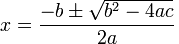Posted in Science & Nature

Anyone who has studied mathematics to some degree will know about algebraic equations. An algebraic equation is an equation that can be solved to find the unknown value of x. A quadratic equation is an algebraic equation with , or in other words has two valid solutions to x. Generally speaking, a quadratic equation can be expressed in the following fashion: ax² + bx + c = 0. a, b and c are constants and the equation can be solved to find x. A quadratic equation is definitely more complicated to solve compared to a linear equation and it can be solved using various means and applications such as factorisation. As these methods are learnt in school and this Encyclopaedia is technically not a mathematics textbook, such methods will not be delved into.

If you have not learnt it already, there is a shortcut method to solving quadratic equations: the quadratic formula. This formula can easily find x if you simply substitute in the values for a, b and c. Of course this formula only works if the solutions are real numbers. The quadratic formula is as follows:As you can see, because of the ± sign, the formula can be used to find both solutions to a quadratic equation. Even without factorising, it can find the answer as long as you substitute numbers into it on a calculator, making maths class very easy. However, as mentioned above the Encyclopaedia of Absolute and Relative Knowledge is not a mathematics textbook and one should instead learn properly from their teacher, not using the formula until they have been taught it properly.